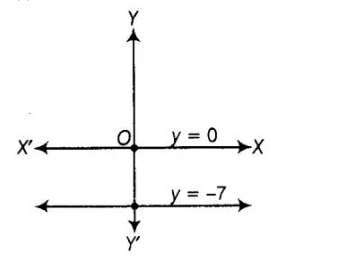# The pair of equations y = 0 and

Question:

The pair of equations y = 0 and y = – 7 has

(a) one solution

(b) two solutions

(c) infinitely many solutions

(d) no solution

Solution:

(d) The given pair of equations are $y=0$ and $y=-7$.By graphically, both lines are parallel and having no solution